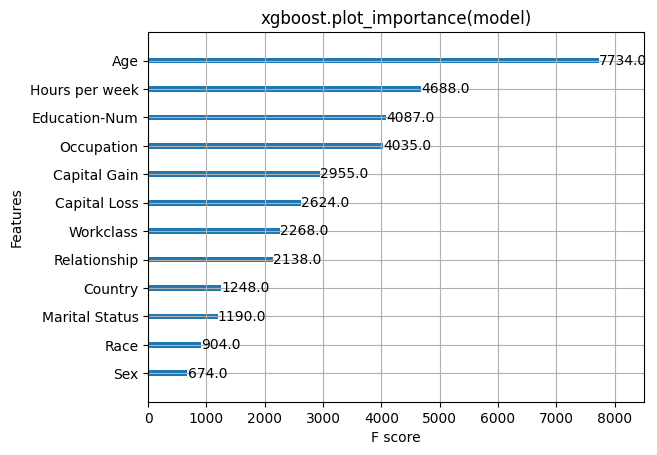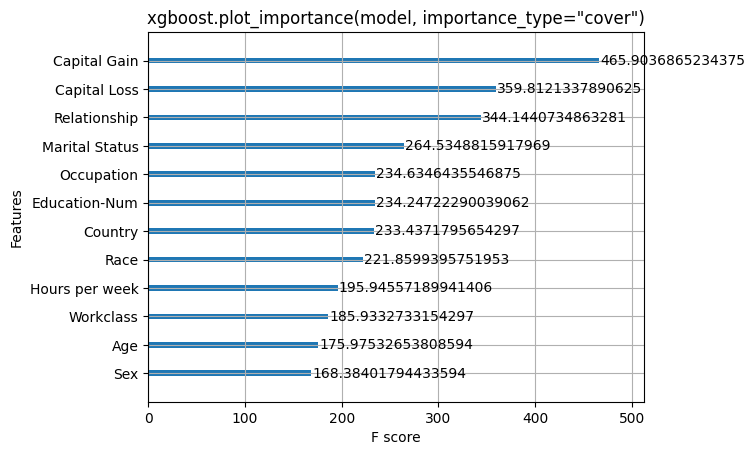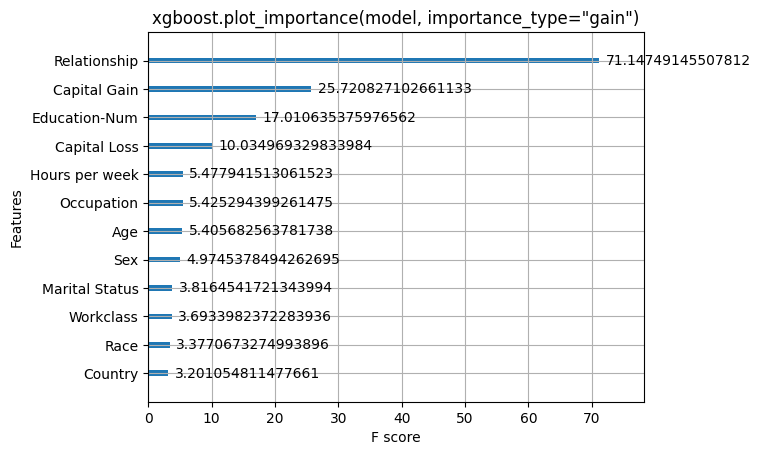# Census income classification with XGBoost

This notebook demonstrates how to use XGBoost to predict the probability of an individual making over \$50K a year in annual income. It uses the standard UCI Adult income dataset. To download a copy of this notebook visit github.

Gradient boosting machine methods such as XGBoost are state-of-the-art for these types of prediction problems with tabular style input data of many modalities. Tree SHAP (arXiv paper) allows for the exact computation of SHAP values for tree ensemble methods, and has been integrated directly into the C++ XGBoost code base. This allows fast exact computation of SHAP values without sampling and without providing a background dataset (since the background is inferred from the coverage of the trees).

Here we demonstrate how to use SHAP values to understand XGBoost model predictions.

:

from sklearn.model_selection import train_test_split
import xgboost
import shap
import numpy as np
import matplotlib.pylab as pl

# print the JS visualization code to the notebook
shap.initjs():

X,y = shap.datasets.adult()

# create a train/test split
X_train, X_test, y_train, y_test = train_test_split(X, y, test_size=0.2, random_state=7)
d_train = xgboost.DMatrix(X_train, label=y_train)
d_test = xgboost.DMatrix(X_test, label=y_test)


## Train the model

:

params = {
"eta": 0.01,
"objective": "binary:logistic",
"subsample": 0.5,
"base_score": np.mean(y_train),
"eval_metric": "logloss"
}
model = xgboost.train(params, d_train, 5000, evals = [(d_test, "test")], verbose_eval=100, early_stopping_rounds=20)

     test-logloss:0.546553
Will train until test-logloss hasn't improved in 20 rounds.
   test-logloss:0.363534
   test-logloss:0.317875
   test-logloss:0.300772
   test-logloss:0.291723
   test-logloss:0.286685
   test-logloss:0.283553
   test-logloss:0.281447
   test-logloss:0.280105
   test-logloss:0.279278
  test-logloss:0.27878
  test-logloss:0.278399
  test-logloss:0.278096
Stopping. Best iteration:
  test-logloss:0.277909



Here we try out the global feature importance calcuations that come with XGBoost. Note that they all contradict each other, which motivates the use of SHAP values since they come with consistency gaurentees (meaning they will order the features correctly).

:

xgboost.plot_importance(model)
pl.title("xgboost.plot_importance(model)")
pl.show():

xgboost.plot_importance(model, importance_type="cover")
pl.title('xgboost.plot_importance(model, importance_type="cover")')
pl.show():

xgboost.plot_importance(model, importance_type="gain")
pl.title('xgboost.plot_importance(model, importance_type="gain")')
pl.show()## Explain predictions

Here we use the Tree SHAP implementation integrated into XGBoost to explain the entire dataset (32561 samples).

:

# this takes a minute or two since we are explaining over 30 thousand samples in a model with over a thousand trees
explainer = shap.TreeExplainer(model)
shap_values = explainer.shap_values(X)


### Visualize a single prediction

Note that we use the “display values” data frame so we get nice strings instead of category codes.

:

shap.force_plot(explainer.expected_value, shap_values[0,:], X_display.iloc[0,:])

:

Visualization omitted, Javascript library not loaded!
Have you run initjs() in this notebook? If this notebook was from another user you must also trust this notebook (File -> Trust notebook). If you are viewing this notebook on github the Javascript has been stripped for security.

### Visualize many predictions

To keep the browser happy we only visualize 1,000 individuals.

:

shap.force_plot(explainer.expected_value, shap_values[:1000,:], X_display.iloc[:1000,:])

:

Visualization omitted, Javascript library not loaded!
Have you run initjs() in this notebook? If this notebook was from another user you must also trust this notebook (File -> Trust notebook). If you are viewing this notebook on github the Javascript has been stripped for security.